Subsections

# Lennard-Jones potential revisited

Before we proceed developing program for the MD simulations of particles interacting via the Lennard-Jones (or any other) potential we have to derive the expression for the force, and for the characteristic time scale, to determine the integration time step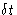.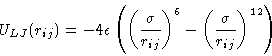(9)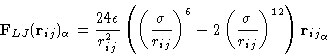(10)

The time scale can be determined by calculating the characteristic oscillations frequency for two particles interacting via the Lennard-Jones potential. Consider two particles of the same mass that are bound together by the Lennard-Jones potential with parametersand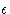. Distance between them is close to the equilibrium distance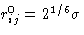.As the force is central we can consider a one-dimensional problem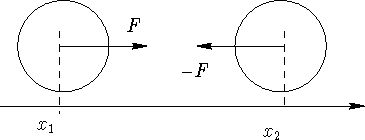If we assume, that they oscillate around the rij0 with a very small amplitude, we can expand the potential energy around rij0 and find for the force: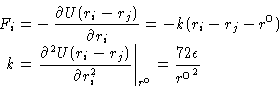(11)

Equations of motion and characteristic frequency then are: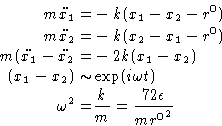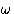is the characteristic frequency of the oscillations, which sets a time scale to choose the time step. Usually for the Verlet algorithm.

## Potential cut-off in MD

One technical remark should be made before we proceed to the program code. Same as in Monte-Carlo, the potential cut-off is used in the MD simulations. Two important consequences:
• energy has a discontinuity at the cut-off distance rc, and
• force is then undefined at rc

This introduces the error in the numerical integration and energy conservation. The cure is to use spline, that would make the potential and force continuous. This, however precludes the cut-off correction or makes it difficult. Simple way to cope with it is just to ignore the error in the force if the rc is large enough, and to use the shifted potential energy U = U - U(rc) to control the energy conservation.Next: Quiz Up: Molecular Dynamics I Previous: Numerical Integration. Finite Difference

© 1997 Boris Veytsman and Michael Kotelyanskii
Tue Nov 25 22:38:03 EST 1997